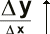Rational Functions

Modeling Representation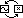Just as polynomials are composed of power functions, rational functions are the ratio of polynomials. A rational function is just one polynomial divided by another.

Predictably, rational functions share many behaviors with polynomials and power functions. However, just as polynomials exhibit behaviors that power functions would never dream of, rational functions exhibit behaviors unlike any polynomial.

To understand the behavior of a rational function, it is enough to consider the long term and short term behaviors of the two polynomials of which it is composed.

In the long term, as we have noted, polynomials are dominated by a single power function. Correspondingly, the long term behavior of rational functions is essentially the ratio of two power functions. The ratio of two power functions is itself a power function. Thus, just like polynomials, rational functions behave simply – like power functions – in the long term.

The short term behavior of rational functions is similarly determined by the short term behavior of the two component polynomials. It is convenient to measure the short term behavior of a polynomial in terms of its roots – the places where its output is zero and the graph crosses the x-axis. A polynomial's characteristic wiggling may produce many crossings of the x-axis in the short term. When the polynomial in the numerator of a rational function has a root, so does the entire rational function. (Zero over anything is zero.) When the polynomial in the denominator of a rational fuction has a root, the rational function becomes undefined. (Anything over zero is undefined.)

Ironically, it is exactly the places where rational functions become undefined that distinguish them. The reason is that, just before and just after becoming undefined, in the intervals around their failings, rational functions leap suddenly towards infinity and away from the behaviors of other functions. These places are called singularities.

 Rational functions model data with singularities.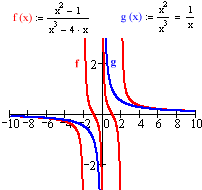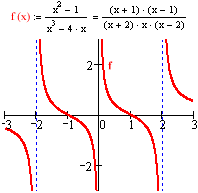Here are some places to look for singularities:

• A lighthouse beam moving along a shoreline. (velocity vs. angle)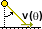• Pressure of a compressed gas. (pressure vs. distance)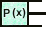• Repellant forces at close range. (force vs. distance)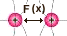• Anything per something getting small. (y vs. x)